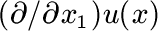Approximation of Solutions and Derivatives for Singularly Perturbed Elliptic Convection-Diffusion Equations

Grigorii I. Shishkin

In this paper we consider mesh approximations of a boundary value problem for singularly perturbed elliptic equations of convection-diffusion type on a strip. To approximate the equations, we use classical finite difference approximations on piecewise-uniform meshes condensing in a neighbourhood of the boundary layer. The approximation errors of solutions and derivatives are analysed in the r-metric. In this metric the error of a solution is defined by an absolute error, while the error of its derivative, i.e. the derivative in the direction across of the boundary layer, is defined by the relative error in that part of the domain where the derivative is large, and by the absolute error in the remainder part of the domain. It is shown that in the class of meshes, whose stepsize in the boundary layer does not decrease with moving away from the outflow boundary, there are no meshes on which the scheme converges e-uniformly in the r-metric. We establish conditions, imposed on the distribution of the nodes of piecewise uniform meshes, under which the scheme converges in the r-metric e-uniformly up to a logarithmic factor.